# Reasoning Tips and Tricks - Order and RankingThe ranking is generally defined as the arrangement of persons/objects in a particular order and to determine the position of a person/object in a row/queue.

This section might be one of the easiest sections in the Reasoning part. There is a maximum of 5 questions which can be expected in a form of a puzzle where we need to determine the order in which the persons/objects are arranged (or) a minimum of 1-2 individual questions in which we are required to find the position of a person/object.

For simplification of understanding, I have sub-divided this topic into various cases.

## Position based Problems

### Type-1:

To find out the position of a person in the row from L.H.S/R.H.S (To find out the position of a person in a row i.e., either from top/bottom)
The position of a person from L.H.S/top = Total number of people in a row + 1 – Position of the same person from R.H.S/bottom.
The position of a person from R.H.S/bottom = Total number of people in a row + 1 – Position of the same person from L.H.S/top.

#### Example-1:

There are 12 persons in a row. The position of Vipul is 7th from left. What is his position from the right end?
Solution
→Total number of people = 12
→Position of Vipul from the left end = 7th
→Position of Vipul from the right end = Total number of people in a row + 1 – position of Vipul from →the left end
= 12 +1 – 7
= 6
→Therefore, Vipul is 6th from the right end#### Example-2:

The position of Sita is 11th from the top in a column of 50 students. What is her position from the bottom?
Solution
→Total number of people = 50
→The position of Sita from top = 11th
→The position of side from bottom = (Total number of people + 1 – position of Sita from top)
= 50 + 1 – 11
= 40
ஃTherefore, the position of Sita from the bottom = 40th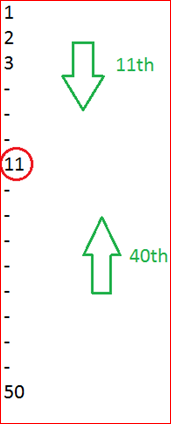### TYPE 2:

To find out the total number of persons in the row
CASE 1: Here the position of a person from L.H.S as well as R.H.S is already given, and we are asked to find out the number of persons in the row
A total number of persons in a row = Position of a person from L.H.S/top + Position of the same person from R.H.S/bottom – 1.

#### EXAMPLE-3:

Vishal is standing in a row. His position from the top is 6th and his position from the bottom is 12th.How many people are there in a row?
Solution
→Position of Vishal from both sides is provided to us.
So,
→A total number of persons in a row = Position of Vishal from Top + Position of Vishal from →Bottom – 1.
→Total number of people in a row = 6th + 12th – 1
= 17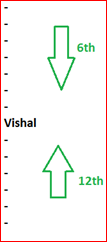CASE-2:
We need to find out the total number of a person in the row when - Position of one person is given from the left end and of the other is given from the right end. The number of persons in between them is also given.

#### Example-4:

In a row, the position of Rahul is 10th from left in a row. Kajol is 17th from the right. Prem is ahead of Rahul by four positions and between Prem and Kajol, there are 6 persons between them. What is the total number of persons in the row?
Solution
→Position of Rahul = 10th from left
→Position of Kajol = 17th from right
→Position of Prem from Rahul = 4
→Persons between Prem and Kajol =6
→Total number of people in a row = 10+17+4+6 = 37CASE-3: Position of one is given from the left end and of the other is given from the right end. The position of persons in between them is also given.

#### Example-5:

The position of Farhaan is 15th from left in a row. Sushant is 20th from the right end. There are 6 persons in between them. What is the total number of persons in the row?
Solution
→Position of Farhaan = 15th from the left end
→Position of Sushant = 20th from right end
→Persons in between them = 6
→Total number of people in the row = 15 + 20 + 6 = 41CASE-4: Position of one is given from the left end and of the other is given from the right end. The total number of persons in the row is also given. We have to determine the number of persons in between them.

#### Example-6:

The position of Sushma is 7th from the left in a row. Bhisma is 23rd from the right end. There is total of 57 persons in the row. What is the total number of persons in between them?
Solution
→Position of Sushma = 7th from left
→Position of Bhisma = 23rd from right
→Total number of people in the row = 57
→Number of people between Sushma and Bhisma = 57 – ( 23 + 7 ) = 27CASE-5: We need to obtain the total number of persons sitting in a row when two people interchange their position.

#### Example-7:

James is 14th from left and Nancy is 25th from the right. They interchange their positions. Now James is 39th from left and Nancy is 50th from the right. What is the total number of persons in the row?
Solution→As we have discussed earlier, to determine the total number of people, we need the L.H.S and R.H.S value of the same person.
→If we consider position-1, we observe that James is 14th from left and Nancy is 50th from the right. →Nancy is 50th from right after interchanging their positions. This implies that the initial position of →James from the right end was 50th.
→A total number of persons in a row = Position of James from left + Position of James from right – 1.
14 + 50 – 1 = 63
→The Same result is obtained if we look at the position-2.
→Nancy was 25th from right and now at the same position, James is 39th from left. So, we simply add up both the rankings i.e., from left and right and reduce by 1.
25 + 39 – 1 = 63
→Therefore, the total number of people in the row = 63.

## Practice Exercise with Solution

Q1) Rohit is 7 ranks ahead of Karan in a class of 39. If Karan’s rank is 17th from the last, what will be Rohit’s rank from the start?
a) 14th
b) 15th
c) 16th
d) 17th
e)None of these

Solution
→Given Karan is 17th from last
→Rohit is 7 ranks ahead of Karan. Since, the position of Karan is given from the last, Rohit rank from last is
17 + 7 = 24
→Position of Rohit from the start = Total strength of the class + 1 - Position of Rohit from the bottom
= 39 + 1 – 24
=16th

Q2) In a class of 39 students, the ratio of boys and girls is 2:1. Akruthi ranks 15th among all the students from top and 8th among girls from the bottom. How many boys are there below Akruthi?
a) 16
b) 17
c) 15
e) None of these
SolutionThere are 26 boys and 13 girls→ Among 13 girls, 7 girls are above Akruthi. So, the remaining girls should be below her i.e., ( 13 – 8 ) = 5 girls are below her.
→ Since there are only 7 girls above Akruthi, the remaining 7 places are occupied by boys. So, the remaining boys will be below Akruthi.
→ Below Akruthi, there are 39-15 = 24 students. Among those 24 students, the number of boys below Akruthi is
24 – 7 = 17

Q3) In a queue, Roshan is 14th from the front and Jeelani is 17th from the end, while Aysha is in between them. If Roshan is ahead of Jeelani and there are 48 persons in the queue. How many persons are there between Roshan and Aysha?
a) 8
b) 7
c) 6
d) 7
e) None of these
Solution→The number of people between Roshan and Jeelani = 48 – ( 14 + 17 ) = 17 person
→Now, Aysha is exactly between Roshan and Jeelani.
→Therefore, there are 8 persons between Roshan and Aysha.

Q4) In a row of 40 boys when Kushal was shifted to his left by 4 places, his place from the left end of the row became 10. What is the position of Suraj from the right end of the row, if Suraj was three places to the right of Kushal’s original position?
a) 22
b) 23
c)25
d)24
e) None of these
Solution
→On shifting 4 positions to the left, Kushal is 10th from the left end.
→So, initially, he was at 14th position from the left end, which implies that Suraj is at 17th position from the left end.
→The position of Suraj from the right end = Total number of boys + 1 – Position of Suraj from left end
= 40 + 1 – 17 = 24Q5) In a row of 25 children facing South, R is 16th from the right end and B is 18th from the left end. How many children are there between R and B?
a) 2
b) 4
c)3
e) None
Solution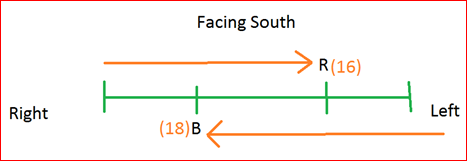→To determine the number of people between B and R, = Total number of people – ( B’s position + R’s position ) =25 – ( 18 + 16 ) = - 9
→Since the number of people can never be negative. Let us find out the position of B and R from the other end.
→Position of R from L.H.S = Total number of students + 1 – Position of R from R.H.S = 25 + 1 – 16 = 10
→Position of B from the R.H.S = Total number of students + 1 – Position of B from L.H.S = 25 + 1 – 18 = 8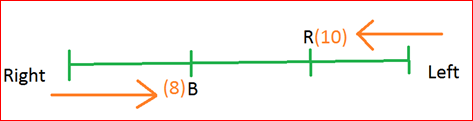→Number of students between B and R = Total number of students – ( B’s position + R’s position )
= 25 – ( 10 + 8 )
= 7
Q6) In a row of 35 children, M is 15th from the right end and there are 10 children between M and R. What is R’s position from the left end of the row?
a) 15th
b) 5th
c)30th
e) None
Solution
→If we observe the question clearly, we notice that there are two possible cases
→In one case, R might be right of M and in another case, R might be left of M.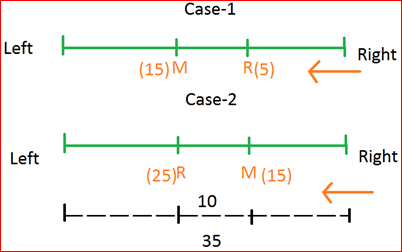→If R is towards the right side of M, then it will be in 5th position from the right end.
→Position of R from L.H.S = 35 + 1 – 5 =31st
→If R is towards the left side of M, then it will be on 25th position from the right end.
→Position of R from L.H.S = 35 +1 – 25 =11th
→Since R can be either 31st or 11th position from the left end. Option (d) is the correct option.

Q7) In a row of 40 children, Q is 14th from the left end and there are 16 children between Q and M. What is M’s position from the right end of the row?
a) 11th
b) 10th
c) 30th
e) None
Solution
→Since Q is 14th from left; there is no chance for M to be left of Q.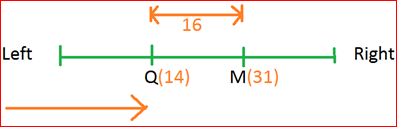→M’s position from the left end = 14 + 16 + 1
= 31st
→M’s position from the right end = Total no of children + 1 – M’s position from the left end
= 40 + 1 – 31
= 10th

Q8) Three persons A, B and C are standing in a queue. There are 5 people between A and B and 8 people between B and C. If there are three people ahead of C and 21 people behind A, then what could be the minimum number of persons in the queue?
a) 27
b) 28
c)40
d) 41
Solution
→As per the given conditions, there are two possible arrangements as shown below:→The number of people in the queue = 21 + 1 + 5 +1 +8 + 3 =39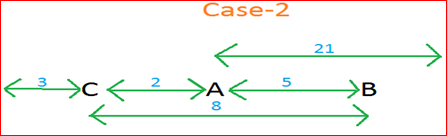→The number of people in the queue = 3 + 1 +2 + 1 + 21 = 28
Clearly, for minimum number of people, we should consider Case-2.

Q9) In a row of children facing North, Sheila is shifted to her right by four places becomes 18th from the right end of the row. Sahil, who is 15th from the left end of the row, is 5th to the left of Sheila. How many children are there in the row?
a) 42
b) 38
c) 41
d) 39
e) None of these
Solution
→After shifting the position of Sheila, her position is 18th from the right end. So, initially, Sheila was 15th from the right end. Saahil is 5th to the left of Sheila.→Total number of children in a row = 15 + 15 + 4
= 34

Q10) In a row of boys facing North, Rinku is 10th to the left to Pink who is 21st from the right end. If Miku who is 17th from the left end, is 4th to the right of Rinku, then how many boys are there in the row?
b) 44
c) 37
d)43
e) None
Solution
Given,
→Pink is 21st from the right end and Rinku is 10th to the left of Pink
→So, Rinku is 31st (i.e., 21 + 10 ) from the right end.
→Miku is 17th from the left end and 4th to the right of Rinku.
→So, Rinku’s position from the left end with respect to Miku = 14th
→Total number of boys = Position of Rinku from R.H.S + Position of Rinku from L.H.S – 1
= 31 + 14 – 1
= 43

Q11) Unita is 11th from either end of a row of girls. How many girls are there in that row?
a) 19
b) 20
c) 21
d) 22
e) 24
Solution
→Total number of girls in row = Position of Unita from L.H.S + Position of Unita from R.H.S - 1
= 11 + 11 -1
= 21

## Comparison Based Problems

Similar to the above problems, in this set of questions- we are required to find the position of a person/thing in comparison with the position of the remaining persons/things.
These types of questions are mostly asked in the form of puzzles.

Q12) Raja walks slower than Raghu and Raghu walk as fast as Guru and Krishna walk faster than Guru. Who walks the fastest?
a) Raghu
b) Raja
c) Krishna
d) Both Raghu and Guru
Solution(Q13-Q14)
Among A, B, C and D, B is heavier than A and C but C is taller than him. D is not as tall as C while A is shortest. C is not as heavy as A. D is not as tall as C while A is shortest. C is not as heavy as A. D is heavier than B but shorter than him.

Q13) Who is the heaviest?
a) B
b) A
c) D
d) C
e) Cannot be determined

Q14) Who is the tallest?
a) D
b) C
c) Either A or D
d) B
e) Cannot be determined
Solution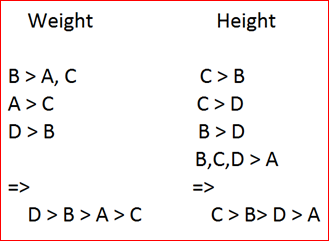Q15) P, Q, R and S are four males. P is the eldest in the group but he is not the poorest. R is the richest but not the eldest, Q is elder than S but he is not the eldest, Q is elder than S. How can the four persons be arranged in descending order of their age and money?
a) PQRS, RPSQ
b) PRQS, RSPQ
c) PRQS, RSQP
d) PRSQ, RSPQ
e) None of these
Solution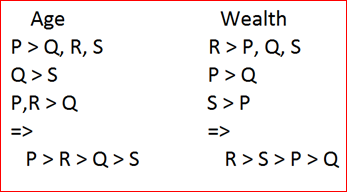(Q16-Q18)
Five people I, J, K, L and M type at different speeds. I type faster than L but slower than J. M types slower than L but is not the slowest. The one who types the second fastest, types at a speed of 75 words/minute. The one who types the second slowest types at the speed of 50 words/minute.

Q16) How many people type slower than I?
a) 4
b) 1
c) 2
d) 3
e) None of these

Q17) On the given information, which of the following statements is true?
a) J types the fastest
b) K types faster than L
c) No statement is true
d) I type at a speed of 50 words/minutes
e) M types faster than only two persons

Q18) Which of the following can be L’s speed?
a) 77 words/minute
b) 60 words/minute
c) 30 words/minute
d) 45 words/minute
e) 85 words/minute
Solution(Q19-Q21)
Among five persons – P, Q, R, S and T, each has different height. Only two persons are shorter than S. T is shorter than S but taller than R. The one who is the second tallest among them is of 158 cm.

Q19) Which of the following statement is definitely true with respect to the given information?
a) R is definitely 150cm
b) P is possibly 153cm
c) T is shorter than S
d) Q is shorter than S
e) None of the above

Q20) Which of the following is the height of Q?
a) 148cm
b) 156cm
c) 152cm
d) 150cm
e) 158cm

Q21)How many persons are shorter than Q?
a) 2
b) 3
c) 4
d) Cannot be determined
e) 1
Solution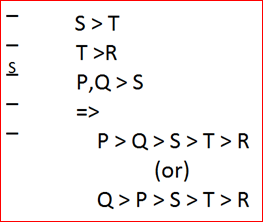→The height of Q will be greater than or equal to 158cm